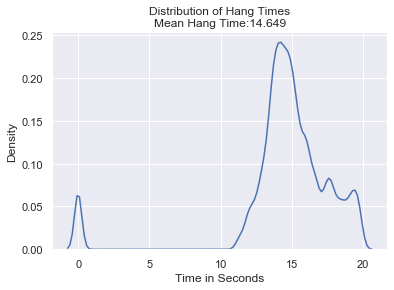### Solving Stochastic Differential Equations in PythonAs you may know from last week I have been thinking about stochastic differential equations (SDEs) recently. As such, one of the things that I wanted to do was to build some solvers for SDEs. One good reason for solving these SDEs numerically is that there is (in general) no analytical solutions to most SDEs.

So I built a solver using the Euler-Maruyama method. There are of course other methods that I intend to build into this project as well. Including a solver for partial differential equations, since you can transform an SDE into an equivalent partial differential equation describing the changes in the probability distribution described by the SDE. This is of course the associated Fokker-Plank equations. The nice thing about that addition is that at the moment with Euler-Maruyama, you start at some initial point with certainty. With a solution for the associated Fokker-Plank equations, you can start with an initial probability distribution instead of a single point of emission.

As an example, of how this solver works, I used it to solve some stochastic kinematic equations. Here is the solution to a projectile shot straight up but subjected to (fairly strong) random updrafts and downdrafts. The black lines represent the maximum and the minimum of the probability distribution of the projectiles vertical position. The soft blue lines are individual trajectories, the bluer the region, the more trajectories pass through that point, and thus the higher the probability of finding the projectile there at that time.

We can also calculate the distribution of hangtimes (now that hangtime is probabilistic as well). When we do that (for a different set of initial conditions than the problem depicted above), you get something that looks like this:

Note that not all trajectories have landed in this scenario, and thus we do have a spike at time t=0. This being the only “zero” that we could find for that particular run (the simulation ran from time t=0 to t=20).